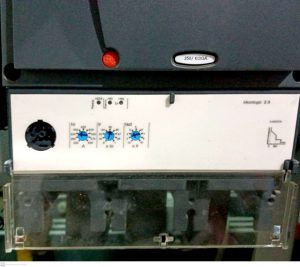# What are Parameters of a Circuit Breakers?

There are different essential parameters of the circuit breakers which necessary to understand. Furthermore, These parameters we often see marked on circuit breakers.

Operating Voltage (Ue)
It is the voltage at which any circuit breaker is designed under normal conditions.

Rated current (In)
This is the maximum value of current that a circuit-breaker, can carry indefinitely at an ambient temperature designed by the manufacturer, without any interruption.

However, These were the parameters which need to understand by every electrical engineer.

Overload Trip (Ir) Setting:

It is used for the continuous ampere rating according to current. It has different set of multiples in the adjustable knob ranging from 0.4 to 1 of nominal or rated current of circuit breaker which can be represented by In.Overload Ir setting of a Circuit Breaker

Ir= multiple *In.
Let suppose we have a circuit breaker having rating of 2000 A which has a current load of 1000A so we will set it 1000A by moving the knob at 0.5 which means
Ir= 0.5*2000
Ir = 1000A